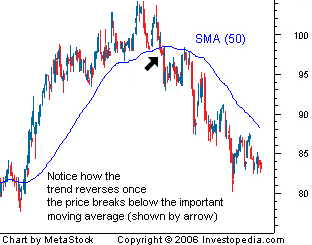# MOJO Day Trading Technical Analysis: Moving Averages

Most traders use moving averages when studying chart patters. A moving average is the average price of a security over a set amount of time. The three most common types of moving averages are simple, linear and exponential.Simple Moving Average (SMA) This is the most common method used to calculate the moving averages. It simply takes the sum of all of the past closing prices over the time period and divides the result by the number of prices used in the calculation. For example, in a 10-day moving average, the last 10 closing prices are added together and then divided by 10.

Linear Weighted Average This moving average is the least common and is used to address the problem of the equal weighting. The linear weighted moving average is calculated by taking the sum of all the closing prices over a certain time period and multiplying them by the position of the data point and then dividing by the sum of the number of periods. For example, in a five-day linear weighted average, today's closing price is multiplied by five, yesterday's by four and so on until the first day in the period range is reached. These numbers are then added together and divided by the sum of the multipliers.

Exponential Moving Average (EMA) This moving average calculation uses a smoothing factor to place a higher weight on recent data points and is regarded as much more efficient than the linear weighted average. Having an understanding of the calculation is not generally required for most traders because most charting packages do the calculation for you. The most important thing to remember about the exponential moving average is that it is more responsive to new information relative to the simple moving average. This responsiveness is one of the key factors of why this is the moving average of choice among many technical traders.

Major Uses of Moving Averages Moving averages are used to identify current trends and trend reversals as well as to set up support and resistance levels.

Moving averages can be used to quickly identify whether a security is moving in an uptrend or a downtrend depending on the direction of the moving average.As you can see here, when a moving average is heading upward and the price is above it, the security is in an uptrend. Conversely, a downward sloping moving average with the price below can be used to signal a downtrend.

Moving average trend reversals are formed in two main ways: when the price moves through a moving average and when it moves through moving average crossovers. The first common signal is when the price moves through an important moving average.For example, when the price of a security that was in an uptrend falls below a 50-period moving average, like here, it is a sign that the uptrend may be reversing.

The other signal of a trend reversal is when one moving average crosses through another.For example, as you can see in here, if the 15-day moving average crosses above the 50-day moving average, it is a positive sign that the price will start to increase.

If the periods used in the calculation are relatively short, for example 15 and 35, this could signal a short-term trend reversal. On the other hand, when two averages with relatively long time frames cross over (50 and 200, for example), this is used to suggest a long-term shift in trend.

Another major way moving averages are used is to identify support and resistance levels. It is not uncommon to see a stock that has been falling stop its decline and reverse direction once it hits the support of a major moving average. A move through a major moving average is often used as a signal by technical traders that the trend is reversing. For example, if the price breaks through the 200-day moving average in a downward direction, it is a signal that the uptrend is reversing.

Moving averages are a powerful tool for analyzing the trend in a security. They provide useful support and resistance points and are very easy to use. The most common time frames that are used when creating moving averages are the 200-day, 100-day, 50-day, 20-day and 10-day. The 200-day average is thought to be a good measure of a trading year, a 100-day average of a half a year, a 50-day average of a quarter of a year, a 20-day average of a month and 10-day average of two weeks.

Moving averages help technical traders navigate the market with day-to-day price movements, giving traders a clearer view of the price trend. Find out more about becoming a ProTrader in the next class of MOJO University. That starts on Tuesday Evening November 19th and runs to December 19th. Get your MOJO on! See more here >

Tags: